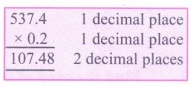Home | | Maths 7th Std | Exercise 1.3 (Multiplication of Decimal Numbers)

# Exercise 1.3 (Multiplication of Decimal Numbers)

7th Maths : Term 3 Unit 1 : Number System : Multiplication of Decimal Numbers : Text Book Back Exercises Questions with Answers, Solution

Multiplication of Decimal Numbers

Exercise 1.3

1. Find the product of the following

(i) 0.5 × 3

5 × 3 = 15

0.5 × 3 = 1.5

(ii) 3.75 × 6

375 × 6 = 2250

3.75 × 6 = 22.50(iii) 50.2 × 4

502 × 4 = 2008

50.2 × 4 = 200.8(iv) 0.03 × 9

3 × 9 = 27

0.03 × 9 = 0.27

(v) 453.03 × 7

45303 × 7 = 317121

453.03 × 7= 3171.21(vi) 4 × 0.7

4 × 7 = 28

4 × 0.7 = 2.8

2. Find the area of the parallelogram whose base is 6.8 cm and height is 3.5 cm.

Solution:

Base of the parallelogram b = 6.8 cm

Height of the parallelogram h = 3.5 cmArea of the parallelogram A = b ×  h sq.units = 6.8 × 3.5 cm2

Area of the parallelogram = 23.80 cm2

3. Find the area of the rectangle whose length is 23.7 cm and breadth is 15.2 cm.

Solution:

Length of the rectangle l = 23.7 cm

Breadth of the rectangle b = 15.2 cmArea of the rectangle A = l × b sq.units

= 23.7 × 15.2 cm2

Area of the rectangle = 360.24 cm2

4. Multiply the following

(i) 2.57 × 10 = 25.7

(ii) 0.51 × 10 = 5.1

(iii) 125.367 × 100 = 12536.7

(iv) 34.51 ×100 = 3451

(v) 62.735 × 100 = 6273.5

(vi) 0.7 × 10 = 7.0

(vii) 0.03 × 100 = 3

(viii) 0.4 ×1000 = 400

5. A wheel of a baby cycle covers 49.7 cm in one rotation. Find the distance covered in 10 rotations.

Solution:

Length covered in 1 rotation = 49.7 cm

Length covered in 10 rotations = 49.7 × 10 cm = 497 cm

6. A picture chart costs ₹ 1.50. Radha wants to buy 20 charts to make an album. How much does she have to pay?

Solution:

Cost of 1 chart = ₹ 1.50

Cost of 20 charts = ₹ 1.50 × 20 = ₹ 30.00Cost of 20 charts = ₹ 30

7. Find the product of the following.

(i) 3.6 × 0.3

36 × 3 = 108

3.6 × 0.3 = 1.08(ii) 52.3 × 0.1

523 × 1 = 523

52.3 × 0.1 = 5.23

(iii) 537.4 × 0.2

5374 × 2 = 10748

537.4 × 0.2 = 107.48(iv) 0.6 × 0.06

6 × 6 = 36

0.6 × 0.06 = 0.036

(v) 62.2 × 0.23

622 × 23 = 14306

62.2 × 0.23 = 14.306.(vi) 1.02 × 0.05

102 × 5 = 510

1.02 × 0.05 = 0.0510

(vii) 10.05 × 1.05

1005 × 105 = 105525

10.05 × 1.05 = 10.5525(viii) 101.01 × 0.01

10101 × l = 10101

101.01 × 0.01 = 1.0101

(ix) 100.01 ×1.1

10001 × 11 = 110011

100.01 × 1.1 = 110.011

Objective type questions

8. 1.07 × 0.1 ________

(i) 1.070

(ii) 0.107

(iii) 10.70

(iv) 11.07

Solution: 107 × 1 = 107

1.07 × 0.1 = 0.107

9. 2.08 × 10 = ________

(i) 20.8

(ii) 208.0

(iii) 0.208

(iv) 280.0

Solution: 208 × 10 = 2080

2.08 × 10 = 20.80 = 20.8

10. A frog jumps 5.3 cm in one jump. The distance travelled by the frog in 10 jumps is

---------

(i) 0.53 cm

(ii) 530 cm

(iii) 53.0 cm

(iv) 53.5 cm

Solution: 53 × 10 = 530

5.3 × 10 = 53.0

Exercise 1.3

1. (i) 1.5 (ii) 22.50 (iii) 200.8 (iv) 0.27 (v) 3171.21 (vi) 2.8

2. 23.80 sq.cm

3. 360.24 sq.cm

4. (i) 25.7 (ii) 5.1 (iii) 12536.7 (iv) 3451 (v) 6273.5 (vi) 7.0 (vii) 3 (viii) 400

5. 497cm

6. ₹ 30

7. (i) 1.08 (ii) 5.23 (iii) 107.48 (iv) 0.036 (v) 14.306 (vi) 0.051 (vii) 10.5525 (viii) 1.0101 (ix) 110.011

Objective type questions

8. (ii) 0.107

9. (i) 20.8

10. (iii) 53.0 cm

Tags : Questions with Answers, Solution | Number System | Term 3 Chapter 1 | 7th Maths , 7th Maths : Term 3 Unit 1 : Number System
Study Material, Lecturing Notes, Assignment, Reference, Wiki description explanation, brief detail
7th Maths : Term 3 Unit 1 : Number System : Exercise 1.3 (Multiplication of Decimal Numbers) | Questions with Answers, Solution | Number System | Term 3 Chapter 1 | 7th Maths